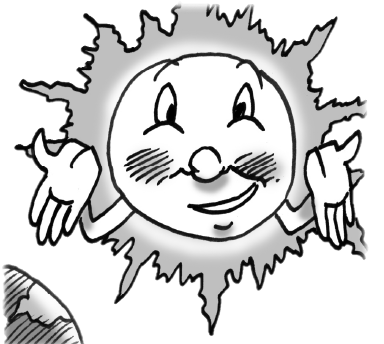Home > CCG > Chapter 11 > Lesson 11.2.3 > Problem11-114

11-114.Perhaps you think the Earth is big? Consider the sun!

1. Assume that the radius of the Earth is $4000$ miles. The sun is approximately $109$ times as wide. Find the sun’s radius.

radius $= (4\text{,}000)(109) ≈ 436\text{,}000$ miles

2. The distance between the Earth and the moon is $238\text{,}900$ miles. Compare this distance with the radius of the sun you found in part (a).

After comparing the two, you should find that the radius of the sun is almost double the distance of the Earth to the moon.

Use $\frac{4}{3}\pi r^3$ to calculate both volumes.

3. If the sun were hollow, how many Earths would fill the inside of it?

$1.295\times10^6$ Earths